### IMO Shortlist 2008 problem G7

Kvaliteta:
Avg: 4,0
Težina:
Avg: 8,5
Let$ABCD$ be a convex quadrilateral with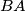$BA$ different from$BC$. Denote the incircles of triangles$ABC$ and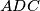$ADC$ by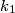$k_{1}$ and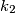$k_{2}$ respectively. Suppose that there exists a circle$k$ tangent to ray$BA$ beyond$A$ and to the ray$BC$ beyond$C$, which is also tangent to the lines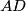$AD$ and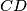$CD$.

Prove that the common external tangents to$k_{1}$ and$k_{2}$ intersects on$k$.

Author: Vladimir Shmarov, Russia
Izvor: Međunarodna matematička olimpijada, shortlist 2008